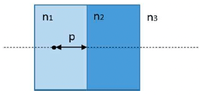# Position of the image of an object placed in water

lorenz0
Homework Statement:
A glass plate of width ##s = 3 cm## with refraction index ##n_2 = 1.5## is in contact with water (##n_1 = 1.33##) on the left and with air on the right. An object is ##p = 10 cm## from the slab. Determine where the image of the object is formed.
Relevant Equations:
##\frac{n_1}{p}+\frac{n_2}{q}=\frac{n_2-n_1}{R}##
I tried using the formula for the refraction of a spherical lens ##\frac{n_1}{p}+\frac{n_2}{q}=\frac{n_2-n_1}{R}## consider each slab as a spherical lens with curvature ##R=\infty## and by doing that I get ##\frac{1.33}{10}+\frac{1.5}{q}=0\Leftrightarrow q\approx -11.3 cm##. Since the piece of glass has a width ##s## I suppose I should count ##11.3 cm## to the left of its rightmost edge, so the object should be ##8.3 cm## to the left of the leftmost edge of the piece of glass i.e. ##1.7 cm## to the right of the original object.

Does this make sense? I had never seen before a problem with "rectangular" lenses and three different materials, so I tried to adapt what I already know to this case. Thanks

#### Attachments

•lens.png
3.7 KB · Views: 23

Homework Helper
Gold Member
I tried using the formula for the refraction of a spherical lens ##\frac{n_1}{p}+\frac{n_2}{q}=\frac{n_2-n_1}{R}##
This is the formula for refraction at a spherical surface. The slab has two surfaces. So, you'll use the formula twice. The image of the first surface serves as the object for the second surface.

•lorenz0 and Lnewqban
lorenz0
This is the formula for refraction at a spherical surface. The slab has two surfaces. So, you'll use the formula twice. The image of the first surface serves as the object for the second surface.
So, if I understand correctly, since from the first application of the equation I get that ##q=-11.3 cm## it means that the first image is formed ##1.3 cm## to the left of the original object, and applying it a second time I get ##\frac{1.5}{11.3+3}+\frac{1}{q}=\frac{1-1.5}{\infty}\Leftrightarrow q\approx -9.53 cm## so the second and final image appears ##9.53 cm## to the left of the rightward surface of the glass which implies, since the glass has a thickness of ##3cm##, that it appears ##3.47 cm## to the right of the original image. Is this correct? Thanks

Homework Helper
Gold Member
I think the problem statement needs clarification:
- object is in water or in air?
- object is 10 cm to the left or the right edge of the plate?

lorenz0
I think the problem statement needs clarification:
- object is in water or in air?
- object is 10 cm to the left or the right edge of the plate?
Looking at the image describing the problem, the object starts inside the water, ##p=10cm## to the left of the leftmost surface of the glass.

Homework Helper
Gold Member
So, if I understand correctly, since from the first application of the equation I get that ##q=-11.3 cm## it means that the first image is formed ##1.3 cm## to the left of the original object, and applying it a second time I get ##\frac{1.5}{11.3+3}+\frac{1}{q}=\frac{1-1.5}{\infty}\Leftrightarrow q\approx -9.53 cm## so the second and final image appears ##9.53 cm## to the left of the rightward surface of the glass which implies, since the glass has a thickness of ##3cm##, that it appears ##3.47 cm## to the right of the original image. Is this correct? Thanks

Your work looks good. It would be better to say that the final image appears ##3.47## cm to the right of the original object, rather than to the right of the original image.

•lorenz0
Homework Helper
Gold Member
Looking at the image describing the problem, the object starts inside the water, ##p=10cm## to the left of the leftmost surface of the glass.
Check. Thanks.

•lorenz0
Homework Helper
Gold Member
Using only Snell's law and small-angle approx. I can corroborate the answer. Do we agree it's a virtual image?

•lorenz0
lorenz0
Using only Snell's law and small-angle approx. I can corroborate the answer. Do we agree it's a virtual image?
Yes, I do agree. Thank you.